# Frank solutions for Class 9 Maths ICSE chapter 26 - Trigonometrical Ratios [Latest edition]

#### Chapters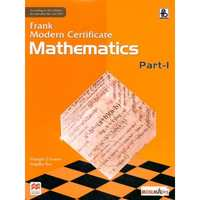## Chapter 26: Trigonometrical Ratios

Exercise 26.1
Exercise 26.1

### Frank solutions for Class 9 Maths ICSE Chapter 26 Trigonometrical Ratios Exercise 26.1

Exercise 26.1 | Q 1.01

In each of the following, one trigonometric ratio is given. Find the values of the other trigonometric.

sinA = (12)/(13)

Exercise 26.1 | Q 1.02

In each of the following, one trigonometric ratio is given. Find the values of the other trigonometric.

cosB = (4)/(5)

Exercise 26.1 | Q 1.03

In each of the following, one trigonometric ratio is given. Find the values of the other trigonometric.

cotA = (1)/(11)

Exercise 26.1 | Q 1.04

In each of the following, one trigonometric ratio is given. Find the values of the other trigonometric.

cose C = (15)/(11)

Exercise 26.1 | Q 1.05

In each of the following, one trigonometric ratio is given. Find the values of the other trigonometric.

tan C = (5)/(12)

Exercise 26.1 | Q 1.06

In each of the following, one trigonometric ratio is given. Find the values of the other trigonometric.

sinB = sqrt(3)/(2)

Exercise 26.1 | Q 1.07

In each of the following, one trigonometric ratio is given. Find the values of the other trigonometric.

cos A = (7)/(25)

Exercise 26.1 | Q 1.08

In each of the following, one trigonometric ratio is given. Find the values of the other trigonometric.

tanB = (8)/(15)

Exercise 26.1 | Q 1.09

In each of the following, one trigonometric ratio is given. Find the values of the other trigonometric.

sec B = (15)/(12)

Exercise 26.1 | Q 1.1

In each of the following, one trigonometric ratio is given. Find the values of the other trigonometric.

cosec C = sqrt(10)

Exercise 26.1 | Q 2.1

In ΔABC, ∠A = 90°. If AB = 5 units and AC = 12 units, find: sinB

Exercise 26.1 | Q 2.2

In ΔABC, ∠A = 90°. If AB = 5 units and AC = 12 units, find: cos C

Exercise 26.1 | Q 2.3

In ΔABC, ∠A = 90°. If AB = 5 units and AC = 12 units, find: tan B.

Exercise 26.1 | Q 3.1

In ΔABC, ∠B = 90°. If AB = 12units and BC = 5units, find: sinA

Exercise 26.1 | Q 3.2

In ΔABC, ∠B = 90°. If AB = 12units and BC = 5units, find: tan A

Exercise 26.1 | Q 3.3

In ΔABC, ∠B = 90°. If AB = 12units and BC = 5units, find: cos C

Exercise 26.1 | Q 3.4

In ΔABC, ∠B = 90°. If AB = 12units and BC = 5units, find: cot C

Exercise 26.1 | Q 4

If sinA = (3)/(5), find cosA and tanA.

Exercise 26.1 | Q 5

If cosB = (1)/(3) and ∠C = 90°, find sin A, and B and cot A.

Exercise 26.1 | Q 6

If sin θ = (8)/(17), find the other five trigonometric ratios.

Exercise 26.1 | Q 7

If tan = 0.75, find the other trigonometric ratios for A.

Exercise 26.1 | Q 8

If sinA = 0.8, find the other trigonometric ratios for A.

Exercise 26.1 | Q 9

If 8 tanθ = 15, find (i) sinθ, (ii) cotθ, (iii) sin2θ - cot2θ

Exercise 26.1 | Q 10.1

In the given figure, PQR is a triangle, in which QS ⊥ PR, QS = 3 cm, PS = 4 cm and QR = 12 cm, find the value of: sin P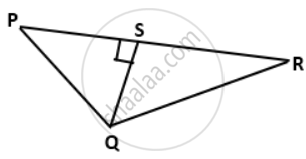Exercise 26.1 | Q 10.2

In the given figure, PQR is a triangle, in which QS ⊥ PR, QS = 3 cm, PS = 4 cm and QR = 12 cm, find the value of: cot2P - cosec2PExercise 26.1 | Q 10.3

In the given figure, PQR is a triangle, in which QS ⊥ PR, QS = 3 cm, PS = 4 cm and QR = 12 cm, find the value of: 4sin2R - (1)/("tan"^2"P")Exercise 26.1 | Q 11.1

In an isosceles triangle ABC, AB = BC = 6 cm and ∠B = 90°. Find the values of cos C

Exercise 26.1 | Q 11.2

In an isosceles triangle ABC, AB = BC = 6 cm and ∠B = 90°. Find the values of cosec C

Exercise 26.1 | Q 11.3

In an isosceles triangle ABC, AB = BC = 6 cm and ∠B = 90°. Find the values of cos2 C + cosec2 C

Exercise 26.1 | Q 12.1

In the given figure, AD is the median on BC from A. If AD = 8 cm and BC = 12 cm, find the value of sin x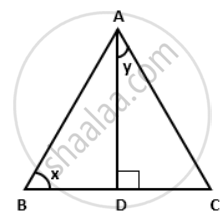Exercise 26.1 | Q 12.2

In the given figure, AD is the median on BC from A. If AD = 8 cm and BC = 12 cm, find the value of cos yExercise 26.1 | Q 12.3

In the given figure, AD is the median on BC from A. If AD = 8 cm and BC = 12 cm, find the value of tan x. cot yExercise 26.1 | Q 12.4

In the given figure, AD is the median on BC from A. If AD = 8 cm and BC = 12 cm, find the value of (1)/("sin"^2 x) - (1)/("tan"^2 x)

Exercise 26.1 | Q 13.1

In a right-angled triangle PQR, ∠PQR = 90°, QS ⊥ PR and tan R =(5)/(12), find the value of sin ∠PQS

Exercise 26.1 | Q 13.2

In a right-angled triangle PQR, ∠PQR = 90°, QS ⊥ PR and tan R =(5)/(12), find the value of tan ∠SQR

Exercise 26.1 | Q 14

In the given figure, ΔABC is right angled at B.AD divides BC in the ratio 1 : 2. Find
(i) ("tan"∠"BAC")/("tan"∠"BAD") (ii) ("cot"∠"BAC")/("cot"∠"BAD")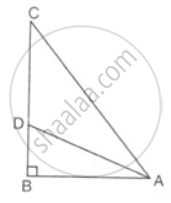Exercise 26.1 | Q 15.1

If sin A = (7)/(25), find the value of : (2"tanA")/"cot A - sin A"

Exercise 26.1 | Q 15.2

If sin A = (7)/(25), find the value of : "cos A" + (1)/"cot A"

Exercise 26.1 | Q 15.3

If sin A = (7)/(25), find the value of : cot2A - cosec2A

Exercise 26.1 | Q 16.1

If cosec θ = (29)/(20), find the value of: cosec θ - (1)/("cot" θ)

Exercise 26.1 | Q 16.2

If cosec θ = (29)/(20), find the value of: ("sec"  θ)/("tan"  θ - "cosec"  θ)

Exercise 26.1 | Q 17.1

In the given figure, AC = 13cm, BC = 12 cm and ∠B = 90°. Without using tables, find the values of: sin A cos A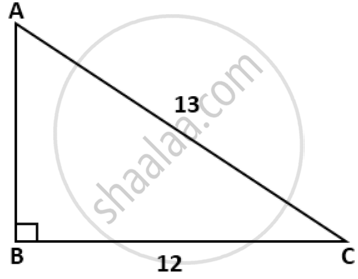Exercise 26.1 | Q 17.2

In the given figure, AC = 13cm, BC = 12 cm and ∠B = 90°. Without using tables, find the values of: ("cos A" - "sin A")/("cos A" + "sin A")Exercise 26.1 | Q 18

In tan θ = 1, find the value of 5cot2θ + sin2θ - 1.

Exercise 26.1 | Q 19.1

In the given figure, ∠Q = 90°, PS is a median om QR from P, and RT divides PQ in the ratio 1 : 2. Find: ("tan" ∠"PSQ")/("tan"∠"PRQ")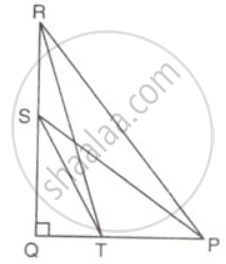Exercise 26.1 | Q 19.2

In the given figure, ∠Q = 90°, PS is a median om QR from P, and RT divides PQ in the ratio 1 : 2. Find: ("tan" ∠"TSQ")/("tan"∠"PRQ")Exercise 26.1 | Q 20.1

In the given figure, AD is perpendicular to BC. Find: 5 cos x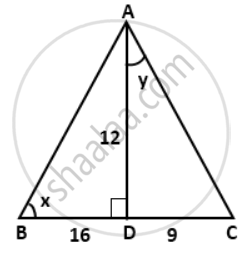Exercise 26.1 | Q 20.2

In the given figure, AD is perpendicular to BC. Find: 15 tan yExercise 26.1 | Q 20.3

In the given figure, AD is perpendicular to BC. Find: 5 cos x - 12 sin y + tan xExercise 26.1 | Q 20.4

In the given figure, AD is perpendicular to BC. Find:
(3)/("sin"  x) + (4)/("cos"  y) - 4 "tan"  y

Exercise 26.1 | Q 21.1

In a right-angled triangle ABC, ∠B = 90°, BD = 3, DC = 4, and AC = 13. A point D is inside the triangle such as ∠BDC = 90°.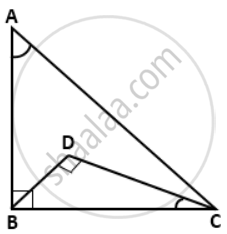Find the values of 2 tan ∠BAC - sin ∠BCD

Exercise 26.1 | Q 21.2

In a right-angled triangle ABC, ∠B = 90°, BD = 3, DC = 4, and AC = 13. A point D is inside the triangle such as ∠BDC = 90°.Find the values of  3 - 2 cos ∠BAC + 3 cot ∠BCD

Exercise 26.1 | Q 22

If 24cosθ = 7 sinθ, find sinθ + cosθ.

Exercise 26.1 | Q 23.1

If 4 sinθ = 3 cosθ, find tan2θ + cot2θ

Exercise 26.1 | Q 23.2

If 4 sinθ = 3 cosθ, find (6sinθ  - 2cosθ )/(6sinθ  + 2cosθ )

Exercise 26.1 | Q 24

If 8tanA = 15, find sinA - cosA.

Exercise 26.1 | Q 25

If 3cosθ - 4sinθ = 2cosθ + sinθ, find tanθ.

Exercise 26.1 | Q 26

If 5cosθ = 3, find the value of (4cosθ - sinθ)/(2cosθ + sinθ)

Exercise 26.1 | Q 27.1

If 4sinθ = sqrt(13), find the value of (4sinθ - 3cosθ)/(2sinθ + 6cosθ)

Exercise 26.1 | Q 27.2

If 4sinθ = sqrt(13), find the value of 4sin3θ - 3sinθ

Exercise 26.1 | Q 28

If 5tanθ = 12, find the value of (2sinθ - 3cosθ)/(4sinθ - 9cosθ).

Exercise 26.1 | Q 29

If 35 sec θ = 37, find the value of sin θ - sin θ tan θ.

Exercise 26.1 | Q 30

If cotθ = (1)/sqrt(3), show that (1 - cos^2θ)/(2 - sin^2θ) = (3)/(5)

Exercise 26.1 | Q 31

If cosecθ = 1(9)/(20), show that (1 - sinθ + cosθ)/(1 + sinθ + cosθ) = (3)/(7)

Exercise 26.1 | Q 32

If b tanθ = a, find the values of (cosθ + sinθ)/(cosθ - sinθ).

Exercise 26.1 | Q 33

If a cotθ = b, prove that ("a"sinθ - "b"cosθ)/("a"sinθ + "b"cosθ) = ("a"^2 - "b"^2)/("a"^2 + "b"^2)

Exercise 26.1 | Q 34

If cotθ = sqrt(7), show that ("cosec"^2θ -sec^2θ)/("cosec"^2θ + sec^2θ) = (3)/(4)

Exercise 26.1 | Q 35

If 12cosecθ = 13, find the value of (sin^2θ  - cos^2θ) /(2sinθ  cosθ) xx (1)/tan^2θ.

Exercise 26.1 | Q 36

If 12 cotθ = 13, find the value of (2sinθ  cosθ)/(cos^2θ - sin^2θ).

Exercise 26.1 | Q 37

If secA = (5)/(4), cerify that (3sin"A" - 4sin^3"A")/(4cos^3"A" - 3cos"A") = (3tan"A" - tan^3"A")/(1 - 3tan^2"A").

Exercise 26.1 | Q 38

If sinθ = (3)/(4), prove that sqrt(("cosec"^2θ - cot^2θ)/(sec^2θ - 1)) = sqrt(7)/(3).

Exercise 26.1 | Q 39

If secA = (17)/(8), verify that (3 - 4sin^2 "A")/(4 cos^2 "A" - 3)= (3 - tan^2"A")/(1 - 3tan^2"A")

Exercise 26.1 | Q 40

If 3 tanθ = 4, prove that sqrt(secθ - "cosec"θ)/(sqrt(secθ - "cosec"θ)) = (1)/sqrt(7).

Exercise 26.1 | Q 41

If tan θ = "m"/"n", show that "m sin θ - n cos θ"/"m sinθ + n cos θ" = ("m"^2 - "n"^2)/("m"^2 + "n"^2)

## Chapter 26: Trigonometrical Ratios

Exercise 26.1## Frank solutions for Class 9 Maths ICSE chapter 26 - Trigonometrical Ratios

Frank solutions for Class 9 Maths ICSE chapter 26 (Trigonometrical Ratios) include all questions with solution and detail explanation. This will clear students doubts about any question and improve application skills while preparing for board exams. The detailed, step-by-step solutions will help you understand the concepts better and clear your confusions, if any. Shaalaa.com has the CISCE Class 9 Maths ICSE solutions in a manner that help students grasp basic concepts better and faster.

Further, we at Shaalaa.com provide such solutions so that students can prepare for written exams. Frank textbook solutions can be a core help for self-study and acts as a perfect self-help guidance for students.

Concepts covered in Class 9 Maths ICSE chapter 26 Trigonometrical Ratios are Concept of Perpendicular, Base, and Hypotenuse in a Right Triangle, Notation of Angles, Trigonometric Ratios and Its Reciprocal, Reciprocal Relations.

Using Frank Class 9 solutions Trigonometrical Ratios exercise by students are an easy way to prepare for the exams, as they involve solutions arranged chapter-wise also page wise. The questions involved in Frank Solutions are important questions that can be asked in the final exam. Maximum students of CISCE Class 9 prefer Frank Textbook Solutions to score more in exam.

Get the free view of chapter 26 Trigonometrical Ratios Class 9 extra questions for Class 9 Maths ICSE and can use Shaalaa.com to keep it handy for your exam preparation